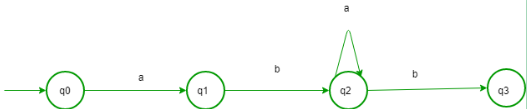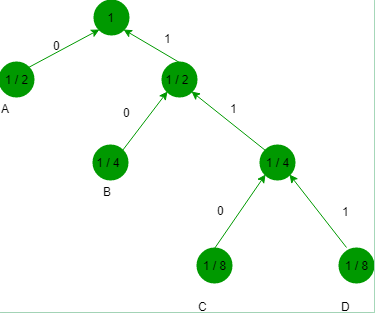# UGC NET COMPUTER SCIENCE SOLVED PAPERS 2014-16 - UGC NET Computer Science Paper 3 July 2016

>>>>>>>>UGC NET Computer Science Paper 3 July 2016

• A

aa*b• B

aa*b• C

aba*b• D

aba*• Option : C
• Explanation :
According to given question, we have transtion: δ(q0, a) = (q1, a, R) δ(q1, b) = (q2, b, R) δ(q2, a) = (q2, a, R) δ(q3, b) = (q3, b, R) We can draw a DFA:Language will be aba*b. So, option (C) is correct.

• A

max I (x; y) p(x)• B

max [H(y) – H(y|x)] p(x)• C

max [H(x) – H(x|y)] p(x)• D

max H(x|y) p(x)• A

2 bits per symbol• B

1.75 bits per symbol• C

1.50 bits per symbol• D

1.25 bits per symbol• Option : B
• Explanation :Bits required per symbol: A - 0 (1 bit) B - 10 (2 bit) C - 110 (3 bit) D - 111 (3 bit) Average number of bits per symbol = 1 * 1 / 2 + 2 * 1 / 4 + 3 * 1 / 8 + 3 * 1 / 8 = 7 / 4 = 1.75. So, option (B) is correct.

• A

Quad Tree• B

Projections• C

Run length coding• D

Chain codes• Option : D
• Explanation :
• Chain codes is used for the boundary representation of an image object
• quad tree is a tree data structure in which each internal node has exactly four children.
• Projection is the presentation of an image on a surface
• Run-length encoding (RLE) is a very simple form of lossless data compression
• So, option (D) is correct.